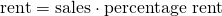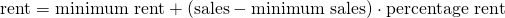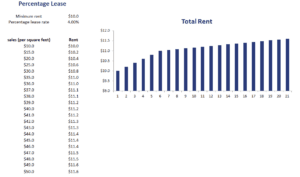# Percentage Lease

A percentage lease or percentage rent is a common type of rent used when leasing retail real estate. A percentage lease requires that retail tenants pay additional rent once sales reach a certain level. On this page, we discuss how a percentage lease is calculated and implement the approach using Excel. The percentage lease calculator is available at the bottom of the page.

## Percentage lease formula

A percentage lease consists of two parts. first, there is the minimum rent. The minimum rent is the amount of rent that has to be paid regardless of the level of sales. Then there is the variable rent. This part of the rent depends on the level of sales. Some percentages rents have a so-called natural breakpoint. In that case, we can easily calculate the percentage rent asIf the percentage rent does not have a natural breakpoint, the calculation is the following## Percentage rent example

Next, let’s consider a simple example to show how we can calculate the rent. The following figure illustrates the calculation in Excel. We assume that the minimum rent is $10 per square foot plus 4% of sales over$40.

In this case, $40 is also the natural break-point. Now, let’s consider different levels of sales. Let’s assume the shop earnings$10, $50, and a$100 per square foot. The results are reported in the figure. Note that, because of the natural break-point, we can calculate the rent directly as a percentage of sales once sales are sufficiently high.### Lease calculator

Want to have an implementation in Excel? Download the Excel file: Percentage Lease

Thus, for low levels of sales, the company only pays the fixed rent. As sales go up, the landlord earns more rent. Clearly, it is very easy to calculate the percentage rent for retail real estate such as shops using an Excel spreadsheet.

## Summary

We discuss the percentage rent, a method used by companies who own retail real estate to benefit from increased popularity and success of the retail real estate they own. The more successful the shopping street or centre, the higher the rent they receive.